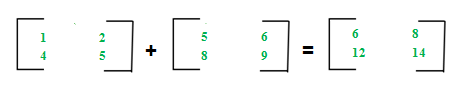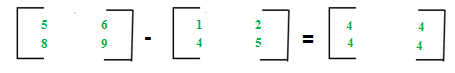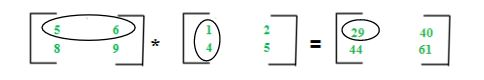# Different Operations on Matrices

For an introduction on matrices, you can refer the following article: Matrix Introduction
In this article, we will discuss various operations on matrices and their properties:

Matrices Addition –
The addition of two matrices A m*n and Bm*n gives a matrix Cm*n. The elements of C are the sum of corresponding elements in A and B which can be shown as:The algorithm for addition of matrices can be written as:

```for i in 1 to m
for j in 1 to n
cij = aij + bij```

Key points:

• Addition of matrices is commutative which means A+B = B+A
• Addition of matrices is associative which means A+(B+C) = (A+B)+C
• The order of matrices A, B and A+B is always the same
• If the order of A and B is different, A+B can’t be computed
• The complexity of addition operation is O(m*n) where m*n is order of matrices

Matrices Subtraction –
The subtraction of two matrices Am*n and Bm*n gives a matrix Cm*n. The elements of C are the difference of corresponding elements in A and B which can be represented as:The algorithm for subtraction of matrices can be written as:

```for i in 1 to m
for j in 1 to n
cij = aij-bij```

Key points:

• Subtraction of matrices is non-commutative which means A-B ≠ B-A
• Subtraction of matrices is non-associative which means A-(B-C) ≠ (A-B)-C
• The order of matrices A, B and A-B is always same
• If order of A and B is different, A-B can’t be computed
• The complexity of subtraction operation is O(m*n) where m*n is order of matrices

Matrices Multiplication –
The multiplication of two matrices Am*n and Bn*p gives a matrix Cm*p. It means number of columns in A must be equal to number of rows in B to calculate C=A*B. To calculate element c11, multiply elements of 1st row of A with 1st column of B and add them (5*1+6*4) which can be shown as:The algorithm for multiplication of matrices A with order m*n and B with order n*p can be written as:

```for i in 1 to m
for j in 1 to p
cij = 0
for k in 1 to n
cij += aik*bkj```

Key points:

• Multiplication of matrices is non-commutative which means A*B ≠ B*A
• Multiplication of matrices is associative which means A*(B*C) = (A*B)*C
• For computing A*B, the number of columns in A must be equal to the number of rows in B
• Existence of A*B does not imply the existence of B*A
• The complexity of multiplication operation (A*B) is O(m*n*p) where m*n and n*p are an order of A and B respectively
• The order of matrix C computed as A*B is m*p where m*n and n*p are order of A and B respectively

## STEM Elearning

We at FAWE have built this platform to aid learners, trainers and mentors get practical help with content, an interactive platform and tools to power their teaching and learning of STEM subjects, more

#### How to find your voice as a woman in Africa

© FAWE, Powered by: Yaaka DN.